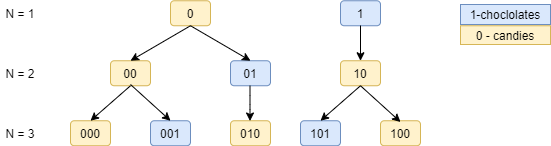# HMAULIS - Editorial

Practice

Contest

Author & Editorialist: Karthikeya

Easy

None

# PROBLEM:

Given an integer N, find the number of distinct N bit binary numbers such that no two consecutive bits are 1 in each of them.

# QUICK EXPLANATION:

By deciding on whether the least significant bit is 0 or 1 for each arrangement, we can see that:

Count(N) = Count(N-1)+Count(N-2)

So this is a Fibonacci series with the first two terms being 2 and 3.

# EXPLANATION:

Let us denote the number of distinct N bit numbers that do not have two consecutive 1's by Count(N).So as we can see in the figure, Count(1) is 2 and Count(2) is 3.

Now to get Count(3) which is for N=3, let us try to add 1 bit at the end of all binary numbers that we have for N=2.

• We can add 0 at the end to 00, 01 and 10 and we get 000,010 and 100 respectively. As we added 0 at the end all the new numbers satisfy the condition that there should not be consecutive 1's. So from here we get Count(2) distinct numbers for N=3 having 0 as the least significant bit. - (1)

• In similar way we cannot add 1 at the end of all the distinct numbers of N=2 as we would get consecutive 1's in few cases. So to add a 1 at the end, the last but one bit should be 0. So we can count these numbers by adding 01 at the end of all distinct numbers of N=2. So from here we get Count(1) disticnt numbers for N=3 having 1 as the least significant bit. - (2)

So from 1 and 2: Count(3) = Count(1) + Count(2).

Similarily for every N we get Count(N) = Count(N-2) + Count(N-1).

So this is a Fibonacci series with the first two terms being 2 and 3.

Hence we precompute the first N numbers of the Fibonacci series with modulus 10^9+7 as A[i] = (A[i-1]+A[i-2])\% 10^9+7 where A=2 and A=3 (A is an array of size 10^6). Then answer each query in O(1).

Time: O(N+T)

Space: O(N)

# SOLUTION:

Setter's Solution

Setters Solution:

``````M = 10**9+7
# initializing array before precomputing
Count = [i+1 for i in range(10**6+2)]

# Precomputation
for i in range(2,10**6+2):
Count[i]= (Count[i-1]+Count[i-2])%M